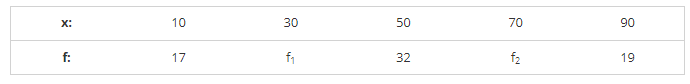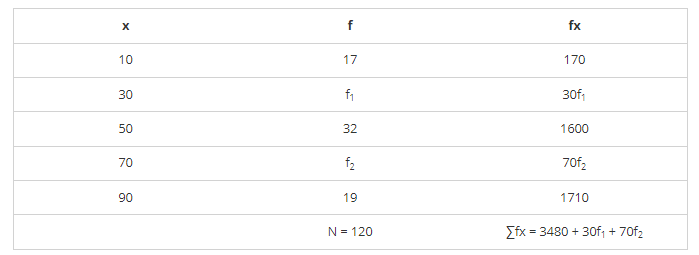# Find the missing frequencies in the following frequency distribution if it is known that the mean of the distribution is 50.

Question:

Find the missing frequencies in the following frequency distribution if it is known that the mean of the distribution is 50.Solution:It is given that

Mean = 50

$\Rightarrow \sum f x / N=50$

$\Rightarrow \frac{3480+30 \mathrm{f}_{1}+70 \mathrm{f}_{2}}{\mathrm{~N}}=50$

$\Rightarrow 3480+30 f_{1}+70 f_{2}=50 \times 120$

$\Rightarrow 30 \mathrm{f}_{1}+70 \mathrm{f}_{3}=6000-3480$

$\Rightarrow 10\left(3 f_{1}+7 f_{2}\right)=10(252)$

$\Rightarrow 3 \mathrm{f}_{1}+7 \mathrm{f}_{2}=252 \cdots(1)[\because$ Divide by 10$]$

And N = 20

$\Rightarrow 17+f_{1}+32+f_{2}+19=120$

$\Rightarrow 68+f_{1}+f_{2}=120$

$\Rightarrow f_{1}+f_{2}=120-68$

$\Rightarrow f_{1}+f_{2}=52$

Multiply with 3 on both sides

$\Rightarrow 3 f_{1}+3 f_{2}=156 \cdots(2)$

Subtracting equation (2) from equation (1)

$\Rightarrow 3 f_{1}+7 f_{2}-3 f_{1}-3 f_{2}=252-156$

$\Rightarrow 4 \mathrm{f}_{2}=96$

$\Rightarrow \mathrm{f}_{2}=96 / 4=24$

Put the value of f2 in equation (1)

$\Rightarrow 3 f_{1}+7 \times 24=252$

$\Rightarrow 3 f_{1}=252-168$

$\Rightarrow f_{1}=84 / 3=28$

$\Rightarrow f_{1}=28$